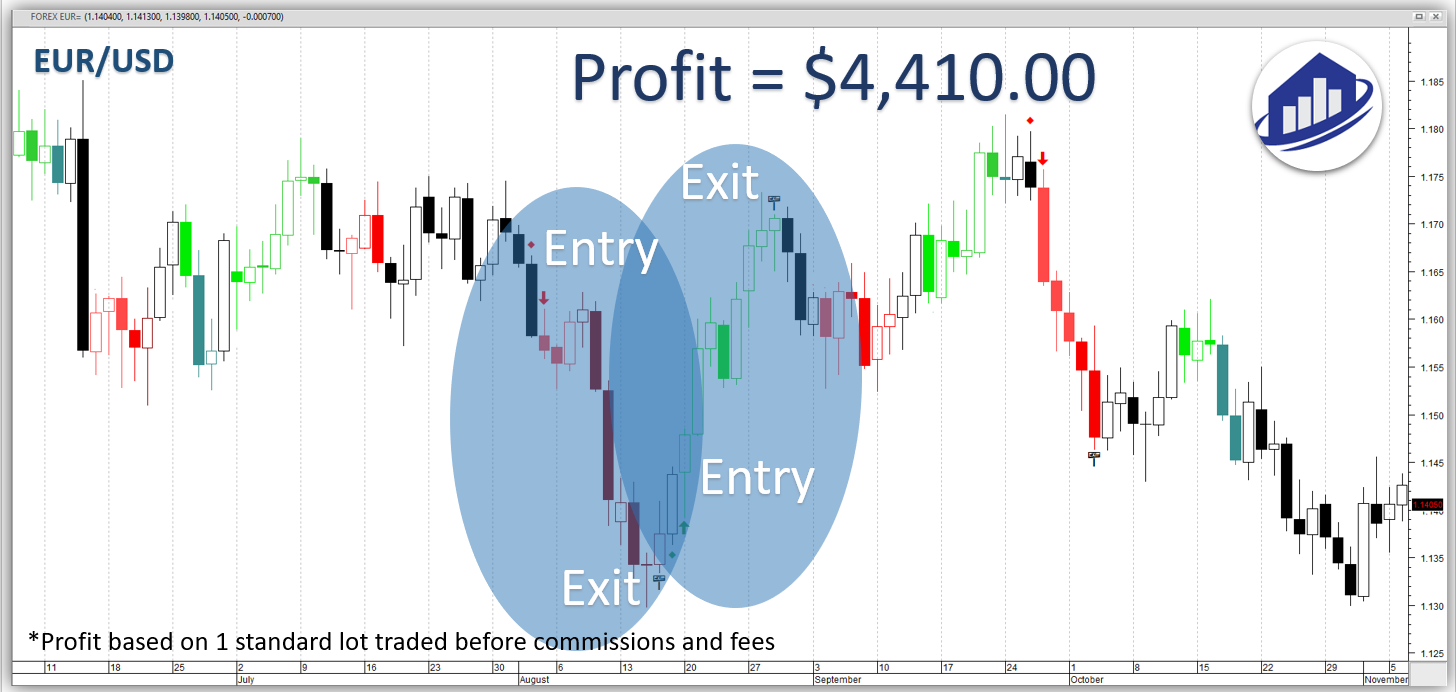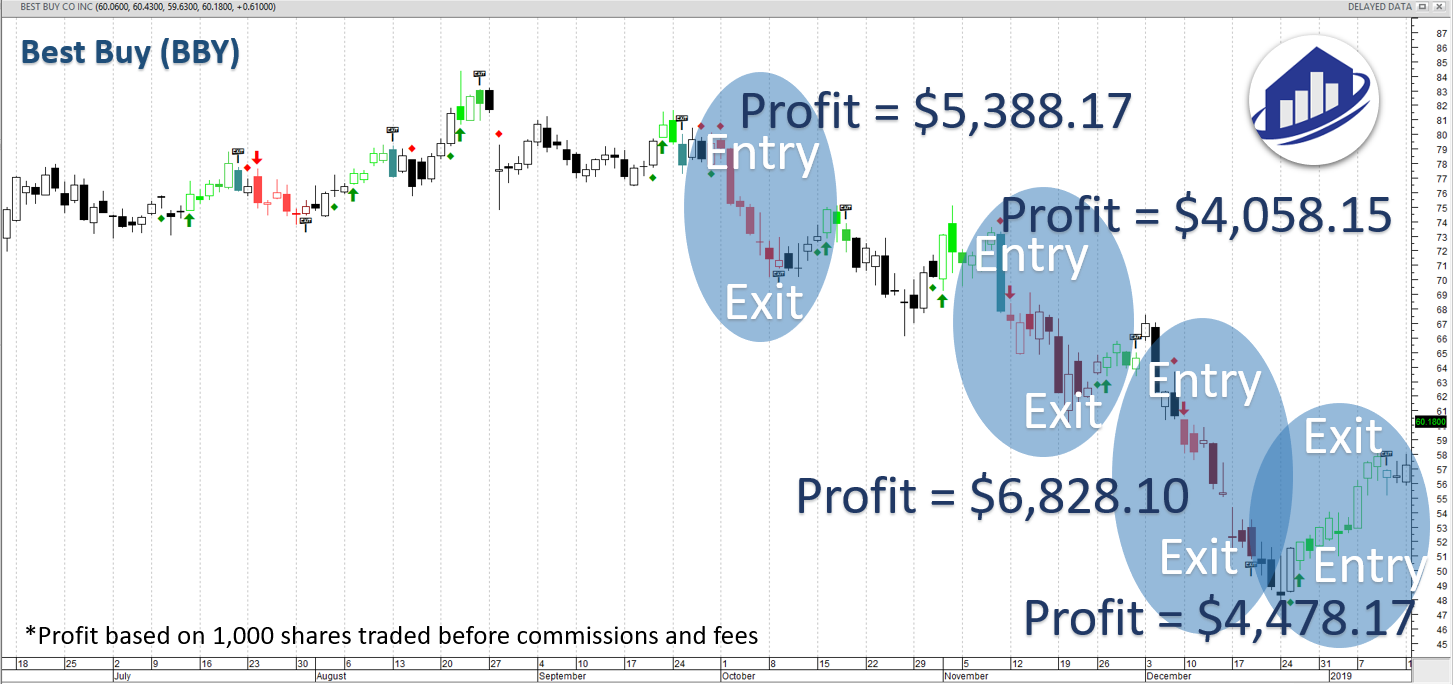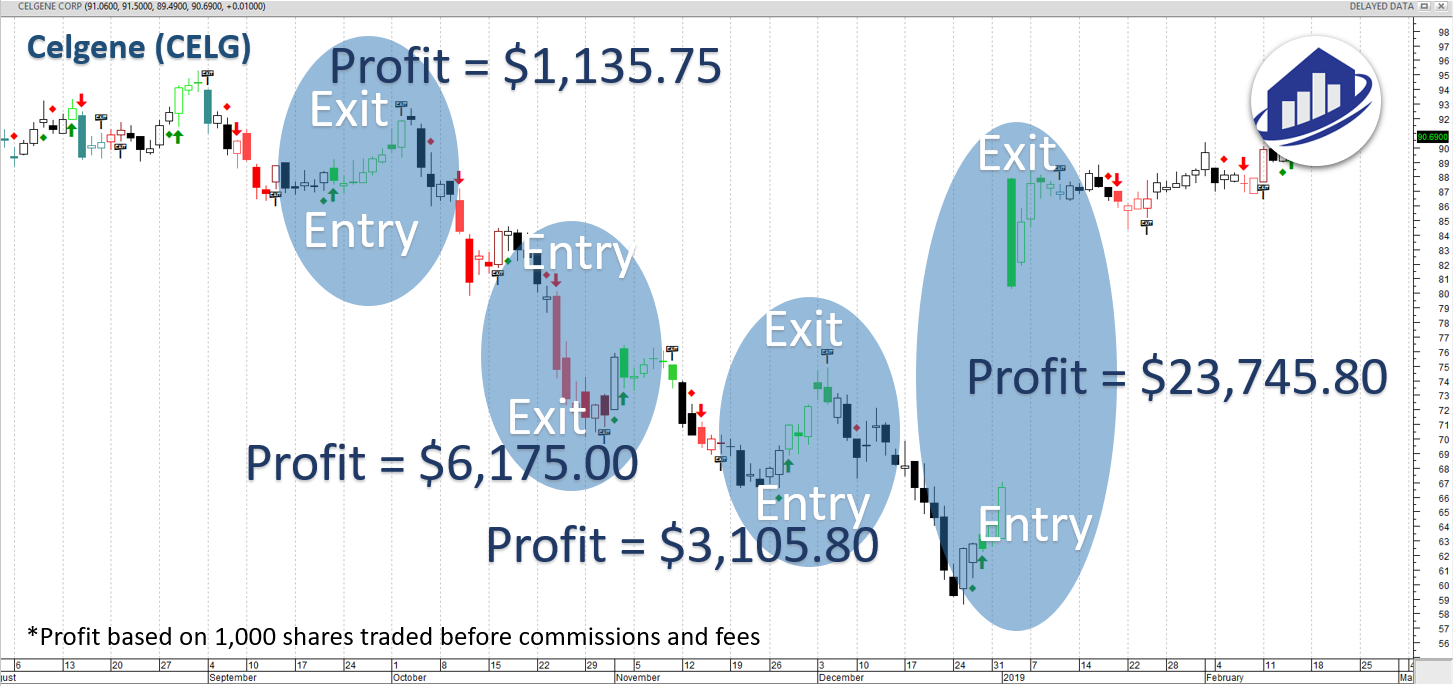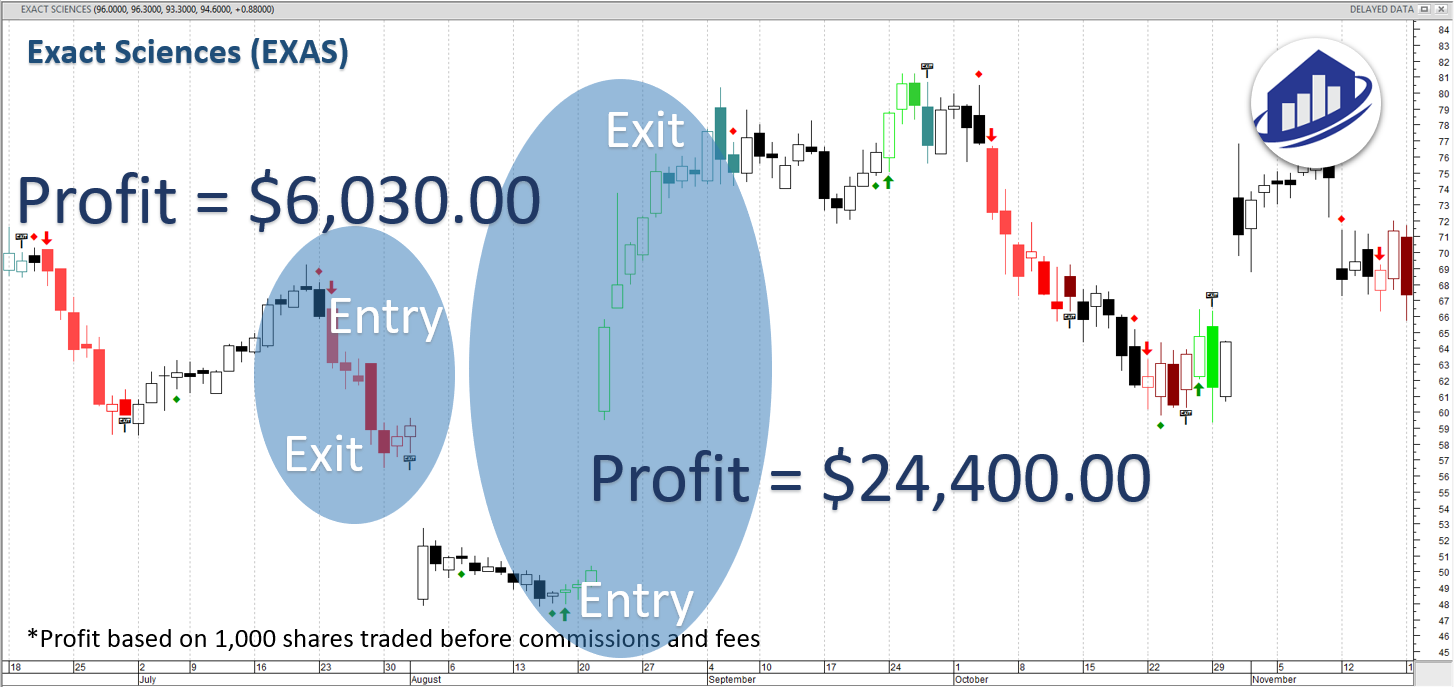Results*The profits shown are hypothetical and represent what is possible if following the signals with the referenced trade size.*The profits shown are hypothetical and represent what is possible if following the signals with the referenced trade size.*The profits shown are hypothetical and represent what is possible if following the signals with the referenced trade size.*The profits shown are hypothetical and represent what is possible if following the signals with the referenced trade size.*The profits shown are hypothetical and represent what is possible if following the signals with the referenced trade size.